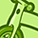# New to Qlik Sense

If you’re new to Qlik Sense, start with this Discussion Board and get up-to-speed quickly.

Announcements
Customer & Partners, DEC. 9, 11 AM ET: Qlik Product & Strategy Roadmap Session: Data Analytics REGISTER NOW
cancel
Showing results for
Did you mean:Contributor III

## How to split a measure in positive and negative values?

Hi Guys,

I have the following measure which calculate a value based on ESTIMATE_AMOUNT now and previous year. The result can be either positive or negative per C_ID. The measure works fine 🙂

-sum(aggr(sum(ESTIMATE_AMOUNT)-above(Sum(ESTIMATE_AMOUNT)), (C_ID, numeric, ascending)),PERIOD_YEAR,numeric, ascending))))

Now to my issue, I want to show the sum of positive & negative values per year separated and stacked in a bar chart over the dimension PERIOD_YEAR. My approach is to create two separate measures, one each for the positive and negative sum.

To make it handy I have made a variable (v_run_off) of the above measure.

I have tried this (for the positive measure) but it doesn't separate over the year, just returns the total;

if(\$(v_run_off)>0, \$(v_run_off))

Now this but it doesn't give me the desired result;

aggr(sum(aggr(if((\$(v_run_off))<0, \$(v_run_off),0),C_ID)),PERIOD_YEAR)

Any guidance on how to solve this would be highly appreciated. RGDS//Peter

1 Solution

Accepted SolutionsContributor III
Author

Hi Anil,

Thank you for your response and for pointing me in the right direction. Yes, you are correct, I made this overly complicated. The trick was to put the if-statement inside the first aggregation (and skip the other aggr :-)). For negative values, here is the solution that works (with an added number formating). Just flip the < for positive values;

num(-sum(aggr(if(ESTIMATE_AMOUNT>above(ESTIMATE_AMOUNT), (ESTIMATE_AMOUNT-above(ESTIMATE_AMOUNT))), (C_ID,(NUMERIC, ASCENDING)), (PERIOD_YEAR,(NUMERIC, ASCENDING)))),'# ##0')

Thanks and RGDS//Peter

2 RepliesMVP

I am not sure why one more aggregate required? Can't you try this

sum(aggr(if(\$(v_run_off)<0, \$(v_run_off),0),C_ID, PERIOD_YEAR))

Before develop something, think If placed (The Right information | To the right people | At the Right time | In the Right place | With the Right context)Contributor III
Author

Hi Anil,

Thank you for your response and for pointing me in the right direction. Yes, you are correct, I made this overly complicated. The trick was to put the if-statement inside the first aggregation (and skip the other aggr :-)). For negative values, here is the solution that works (with an added number formating). Just flip the < for positive values;

num(-sum(aggr(if(ESTIMATE_AMOUNT>above(ESTIMATE_AMOUNT), (ESTIMATE_AMOUNT-above(ESTIMATE_AMOUNT))), (C_ID,(NUMERIC, ASCENDING)), (PERIOD_YEAR,(NUMERIC, ASCENDING)))),'# ##0')

Thanks and RGDS//PeterTags
Community Browser